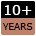Welcome to WebmasterWorld Guest from 54.211.135.32

Forum Moderators: coopster & jatar k

Message Too Old, No Replies

# bitwise left shift differing results

## 32-bit vs 64-bit

#### Nuttzy99

10:03 am on Jun 28, 2006 (gmt 0)

#### New Userjoined:Aug 28, 2003
posts:35

My dev environment is WinXP (32 bit) running PHP 4.3.10. Running this simple compand....

echo (192<<24) ;

...yields a value of -1073741824 which is what I want since that's what most browsers running javascript will yield.

My production/staging environment is a 64-bit AMD Opteron on FreeBSD running PHP 5.0.4. For the same command it yields a value of 3221225472.

I'm guessing it has to do with the 64-bit system. Can anyone tell me how to get my server to match 32-bit systems for this result? I really don't know much about bitwise left shift, but I'm trying to get TEA encryption going.

Thanks,
-Nuttzy

#### Nuttzy99

12:31 pm on June 28, 2006 (gmt 0)

#### New Userjoined:Aug 28, 2003
posts:35

While writing my post I think I figured it out...

define('BIT32_MAX_SIGNED', 2147483648) ; // this is 2 to 31st power (need one bit for sign)

//\$val = 3221225472 ;
\$val = (192<<24) ;
echo \$val . "<br/>" ;
if (\$val > BIT32_MAX_SIGNED)
{
\$val = BIT32_MAX_SIGNED - \$val ;
}
echo \$val . "<br/>" ;

...basically a 32-bit system won't exceed BIT32_MAX_SIGNED, so if we do, then we know it's a 64-bit system and need to adjust. I'll probably need to do the same thing in my javascript encrypt function in case there are any 64-bit browsers out there.

I'm still having problem with my TEA encryption in one case, so I might not have it entirely solved yet.

-Nuttzy

#### Nuttzy99

1:25 pm on June 28, 2006 (gmt 0)

#### New Userjoined:Aug 28, 2003
posts:35

Blah, when val is....

\$val = (192<<24) ;

...it doesn't normalize to the same results as my 32-bit system. I have no idea why. If someone better versed in this stuff has the answer, I'll be happy to learn it.

However, for my needs, I think if I just set my javascript to use unsigned shift (<<<) then I won't have a problem on my 64-bit production system. My 32-bit system is just for development and I can work around it.

Cookie for anyone that solves this ;-)
-Nuttzy

#### Nuttzy99

2:24 pm on June 28, 2006 (gmt 0)

#### New Userjoined:Aug 28, 2003
posts:35

Sorry for all the replies. I made a bad typo in my previous message. It should read...

Blah, when val is....

\$val = (152<<24)

...makes a BIG difference ;-)

-Nuttzy

#### rizal_a

9:32 am on Nov 13, 2006 (gmt 0)

#### New Userjoined:Nov 13, 2006
posts:2

System: The following 2 messages were spliced on to this thread from: http://www.webmasterworld.com/php/3154936.htm [webmasterworld.com] by coopster - 9:30 am on Nov. 14, 2006 (utc -6)

This is regarding Nuttzy99's posts on June 28 2006. The following function will do a 32-bit left shift:

`function _32bitleftshift(\$number, \$steps) {  \$result = 0;  if (strlen(decbin(\$number)) + \$steps <= 31)  {  \$result = \$number << \$steps;  }  else  {  // shift left  \$binary = decbin(\$number).str_repeat("0", \$steps);  // get the last 32 digits  \$binary = substr(\$binary, strlen(\$binary) - 32);  // is the leftmost digit 1, i.e., is it a negative number?  if (\$binary{0} == "1")  {   // get the last 31 digits   \$binary = substr(\$binary, strlen(\$binary) - 31);   // get the 1's complement   \$binary_1s_complement = "";   for (\$i = 0; \$i <= 30; \$i++)   {   \$binary_1s_complement .= (\$binary{\$i} == "0"? "1" : "0");   }   // get the 2's complement   \$binary_2s_complement = decbin(bindec(\$binary_1s_complement) + 1);   \$result = -1 * bindec(\$binary_2s_complement);  }  else  {   \$result = bindec(\$binary);  }  }  return \$result; } `

#### rizal_a

11:55 pm on Nov 13, 2006 (gmt 0)

#### New Userjoined:Nov 13, 2006
posts:2

Whoops ... there's actually a much faster way to find out the 2's complement of any number, which is
``-(pow(2, n) - N)``
where
``n``
is the number of bits and
``N``
is the number for which to find out its 2's complement.

Therefore:

`function leftshift32(\$number, \$steps) {  \$binary = decbin(\$number).str_repeat("0", \$steps);  \$binary = str_pad(\$binary, 32, "0", STR_PAD_LEFT);  \$binary = substr(\$binary, strlen(\$binary) - 32);  if (\$binary{0} == "1")  {   return -(pow(2, 31) - bindec(substr(\$binary, 1)));  }  else  {   return bindec(\$binary);  } } `

#### Hire Expert Members